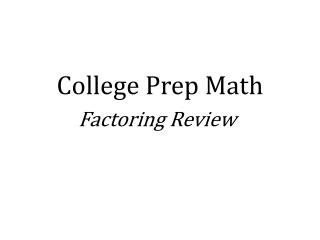DownloadDownload PresentationCollege Prep Math

# College Prep Math

Download Presentation## College Prep Math

- - - - - - - - - - - - - - - - - - - - - - - - - - - E N D - - - - - - - - - - - - - - - - - - - - - - - - - - -
##### Presentation Transcript

1. College Prep Math Factoring Review

2. Any time we have a polynomial the first step before breaking down that polynomial is to Find the greatest common factor. The GCF

3. What is the Greatest Common Factor? The greatest common factor is the greatest factor shared by both terms in a given expression. WHAT YOU TAKE OUT OF ONE TERM, YOU MUST TAKE OUT OF THE OTHER!!

4. Both terms share 6x so we can factor that from each term. We can check to make sure we factored properly by distributing. Our factored solution is YOU HAVE TO MAKE SURE YOU TAKE THE GCF OUT OF BOTH TERMS

5. What is the GCF of Think of the function as 4xxyyy-10xy What is shared in both terms? What is our GCF? Factoring this out of each term will give us what simplified solution? 2,x,y 2xy

6. Difference of Squares The result of a number multiplied by itself. What is a square? (not the shape) Once we have determined that both terms of our polynomial are squares we need to set We have to determine each square and plug that value into the equation.

7. YES Let’s look at Are the given terms perfect squares?... Use the formula Why do we use this formula? When we apply the foil method to combine these factors we end up with.

8. Reverse Foil We want to use the FOIL process, in the opposite order to factor our trinomial. What is the FOIL process? Multiply First terms Multiply Last terms Multiply and Combine Inner terms and Outer Terms

9. Let’s apply our FOIL rules to our given trinomial What two terms do we multiply to give us? What terms can multiply to equal 20? To complete our FOIL process remember we need to combine Outer and Inner terms to = 13x

10. We can add these two terms together because they are like terms to give us 13x and will complete our trinomial. Factoring this will give us…

11. When you are given a polynomial and you are asked to factor, go through the following steps: • Try to factor out a GCF • If we do not have a GCF, look at each term and ask yourself if it is a Perfect Square • If your polynomial is not a perfect square, you need to apply the Reverse Foil Method. (Make sure you check all factors of each term) • If you get to this point and you still can’t factor the polynomial, then indicate It cannot be factored.